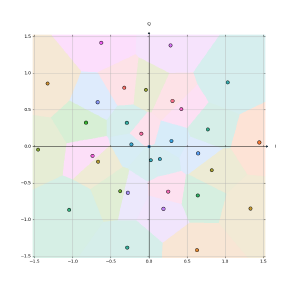matteo
/
constellationnet
Using autoencoders to optimize two-dimensional signal constellations for fiber optic communication systems
You can not select more than 25 topics Topics must start with a letter or number, can include dashes ('-') and can be up to 35 characters long.

#### 99 lines 3.6 KiB Raw Blame History

 `import torch.nn as nn` `import torch` `from .GaussianChannel import GaussianChannel` `from .NormalizePower import NormalizePower` `from . import util` ``` ``` ``` ``` `class ConstellationNet(nn.Module):` ` """` ` Autoencoder network to automatically shape a constellation of symbols for` ` efficient communication over an optical fiber channel.` ` """` ` def __init__(` ` self,` ` order=2,` ` encoder_layers=(),` ` decoder_layers=(),` ` ):` ` """` ` Create an autoencoder.` ` :param order: Order of the constellation, i.e. the number of messages` ` that are to be transmitted, or equivalently the number of symbols whose` ` placements in the constellation have to be learned.` ` :param encoder_layers: Shape of the encoder’s hidden layers. The` ` size of this sequence is the number of hidden layers, with each element` ` being a number which specifies the number of neurons in its channel.` ` :param decoder_layers: Shape of the decoder’s hidden layers. Uses` ` the same convention as `encoder_layers_sizes` above.` ` """` ` super().__init__()` ` self.order = order` ``` ``` ` # Build the encoder network taking a one-hot encoded message as input` ` # and outputting an I/Q vector. The network additionally uses hidden` ` # layers as specified in `encoder_layers`` ` prev_layer_size = order` ` encoder_layers_list = []` ``` ``` ` for layer_size in encoder_layers:` ` encoder_layers_list.append(nn.Linear(prev_layer_size, layer_size))` ` encoder_layers_list.append(nn.ReLU())` ` encoder_layers_list.append(nn.BatchNorm1d(layer_size))` ` prev_layer_size = layer_size` ``` ``` ` encoder_layers_list += [` ` nn.Linear(prev_layer_size, 2),` ` NormalizePower(),` ` ]` ``` ``` ` self.encoder = nn.Sequential(*encoder_layers_list)` ` self.channel = GaussianChannel()` ``` ``` ` # Build the decoder network taking the noisy I/Q vector received from` ` # the channel as input and outputting a probability vector for each` ` # original message. The network additionally uses hidden layers as` ` # specified in `decoder_layers`` ` prev_layer_size = 2` ` decoder_layers_list = []` ``` ``` ` for layer_size in decoder_layers:` ` decoder_layers_list.append(nn.Linear(prev_layer_size, layer_size))` ` encoder_layers_list.append(nn.ReLU())` ` decoder_layers_list.append(nn.BatchNorm1d(layer_size))` ` prev_layer_size = layer_size` ``` ``` ` # Softmax is not used at the end of the network because the` ` # CrossEntropyLoss criterion is used for training, which includes` ` # LogSoftmax` ` decoder_layers_list.append(nn.Linear(prev_layer_size, order))` ``` ``` ` self.decoder = nn.Sequential(*decoder_layers_list)` ``` ``` ` def forward(self, x):` ` """` ` Perform encoding and decoding of an input vector and compute its` ` reconstructed vector.` ` :param x: Original one-hot encoded data.` ` :return: Reconstructed vector.` ` """` ` symbol = self.encoder(x)` ` noisy_symbol = self.channel(symbol)` ` return self.decoder(noisy_symbol)` ``` ``` ` def get_constellation(self):` ` """` ` Extract the symbol constellation out of the trained encoder.` ` :return: Matrix containing `order` rows with the nᵗʰ one being the I/Q` ` vector that is the result of encoding the nᵗʰ message.` ` """` ` with torch.no_grad():` ` return self.encoder(` ` util.messages_to_onehot(` ` torch.arange(0, self.order),` ` self.order` ` )` ``` ) ``` ``` ```